Refer to our Texas Go Math Grade 6 Answer Key Pdf to score good marks in the exams. Test yourself by practicing the problems from Texas Go Math Grade 6 Lesson 5.2 Answer Key Adding Integers with Different Signs.

Reflect

Question 1.
Make a Prediction Predict the sum of – 2 + 2. Explain your prediction and check it using the number line.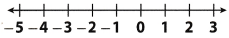The sum of 2 + 2 is 0 because you start at – 2 and move |2| = 2 units right on the number line.
First graph number line with units.
Start at – 2 and move 2 units to the right.
You are 0 units left from 0 on the number line.
The sum is 0.

Number line: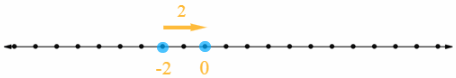The sum of – 2 + 2 is 0 because you start at – 2 and move 2 units right, or in the positive direction.

Question 2.
Make a Prediction Kyle models a sum of two integers. He uses more negative (red) counters than positive (yellow) counters. What do you predict about the sign of the sum? Explain.
The sign of the sum that Kyle models is – (minus).

One positive (yellow) counter and one negative (red) counter form a zero pair, so when you eliminate alt zero pairs, there are some negative (red) counters left.

The sign of the sum that Kyle models is minus, or – because he uses more negative counters.

Model and find each sum using counters.

Question 3.
5 + (- 1) ____________
The sum you want to find is:
5 + (- 1)
Begin with 5 yellow counters to represent 5 (see the picture bellow).
Eliminate zero pairs.
When you remove the zero pairs, there are 4 yellow counters left.

The final result is:
5 + (- 1) = 4There are 5 positive counters and 1 negative and thus 5 + (-1) = 4.

Question 4.
4 + (- 6) ____________
The sum you want to find is:
4 + (- 6)
After 6 red counter to represent adding – 6.
Eliminate zero pairs.
When you remove the zero pairs, there are 2 red counters left.

The final result is:
4 + (- 6) = – 2There are 4 positive and 6 negative counters and thus 4 + (-6) = – 2.

Question 5.
1 + (- 7) ____________
The sum you want to find is:
1 + (- 7)
After 7 red counter to represent adding – 7.
Eliminate zero pairs.
When you remove the zero pairs, there are 6 red counters left.

The final result is:
1 + (- 7) = – 6There are 1 positive and 5 negative counters and thus 1 + (-7) = – 6.

Question 6.
3 + (- 4) ____________
The sum you want to find is:
3 + (- 4)
After 4 red counter to represent adding – 4.
Eliminate zero pairs.
When you remove the zero pairs, there are 1 red counters left.

The final result is:
3 + (-4) = – 1There are 3 positive and 4 negative counters and thus 3 + (-4) = – 1.

Find each sum.

Question 7.
– 51 + 23 = ____________
Find absolute values of both integers and subtract lesser absolute value from greater:
|- 51| – |23| = 28
The final result would be 28 or – 28, depending on signs of given integers.
To find the final result, use the sign of number with greater absolute value:
– 51 + 23 = – 28

The sum is – 28.
Find absolute values, subtract them and add minus if the integer with the greater absolute value was negative.

Question 8.
10 + (- 18) = ____________
Find absolute values of both integers and subtract lesser absolute value from greater:
|- 18| – |10| = 8
The final result would be 8 or – 8, depending on signs of given integers.
To find the final result, use the sign of number with greater absolute value:
10 + (- 18) = – 8

The sum is – 8.
Find absolute values, subtract them and add minus if the integer with the greater absolute value was negative.

Question 9.
13 + (- 13) = ____________
The opposite of an integer is called its additive inverse.
Sum of integer and its additive inverse is 0
so final result is:
13 + (- 13) = 0

The sum is 0 because the sum of an integer and its additive inverse is 0.

Question 10.
25 + (- 26) = ____________
Find absolute values of both integers and subtract lesser absolute value from greater:
|- 26| – |25| = 1
The final result would be 1 or – 1, depending on signs of given integers.
To find the final result, use the sign of number with greater absolute value:
25 + (- 26) = – 1

The sum is – 1.
Find absolute values, subtract them and add minus if the integer with the greater absolute value was negative.

Use a number line to find each sum.

Question 1.
9 + (- 3) = __________First graph number line with units.
Start at 9 and move |- 3| = 3 units in the negative direction (to the left) on the number line.
To find the final result read sum from number line.

Number line: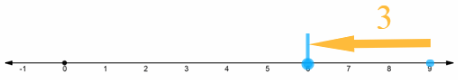The sum is 6 because it is 6 units right from 0 on the number line.

Question 2.
– 2 + 7 = ____________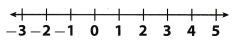First graph number line with units.
Start at – 2 and move |7| = 7 units in the negative direction (to the left) on the number line.
To find the final result, read sum from number line.

Number line: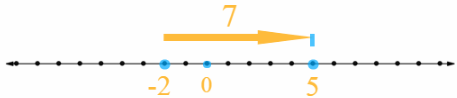The sum is 5 because it is 5 units right from 0 on the number line.

Question 3.
– 15 + 4 = _____________First graph number line with units.
Start at – 15 and move |4| = 4 units in the negative direction (to the left) on the number line.
To find the final result, read sum from number line.

Number line:The sum is – 11 because it is 11 units right from 0 on the number line.

Question 4.
1 + (- 4) = _____________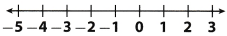First graph number line with units.
Start at 1 and move |-4| = 4 units in the negative direction (to the left) on the number line.
To find the final result, read sum from number line.

Number line:The sum is – 3 because it is 3 units right from 0 on the number line.

Circle the zero pairs in each model. Find the sum.

Question 5.
– 4 + 5 = _____________The sum you want to find is:
– 4 + 5
Start with 4 red counters to represent – 4 and add 5 yellow counters to represent 5.
Find zero pairs and circle them.
When you remove the zero pairs, there is 1 yellow counter left so the sum is:
– 4 + 5 = 1There are 4 negative counters and 5 positive counters and thus – 4 + 5 = 1.

Question 6.
– 6 + 6 = _____________The sum you want to find is:
– 6 + 6
Start with 6 red counters to represent – 6 and add 6 yellow counters to represent 6.
Find zero pairs and circle them.
When you remove the zero pairs, there is 0 yellow counter left so the sum is:
– 6 + 6 = 0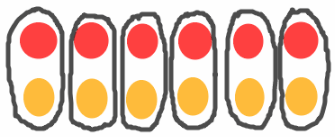There are 6 negative counters and 6 positive counters and thus – 6 + 6 = 0.

Question 7.
2 + (- 5) = _____________The sum you want to find is:
2 + (- 5)
Find zero pairs and circle them.
When you remove the zero pairs, there are 3 red counters left so the sum is:
2 + (- 5) = – 3There are 2 positive counters and 5 negative and thus 2 + (- 5) = – 3.

Question 8.
– 3 + 7 = ______________The sum you want to find is:
– 3 + 7
Find zero pairs and circle them.
When you remove the zero pairs, there are 4 yellow counters left so the sum is:
– 3 + 7 = 4There are 3 negative and 7 positive counters and thus – 3 + 7 = 4

Find each sum.

Question 9.
– 8 + 14 = ______________
Find absolute values and subtract lesser absolute value from greater:
|14| – |- 8| = 6
The final result would be 6 or – 6, depending on signs of given integers.
To find the final result, use the sign of number with greater absolute value:
– 8 + 14 = 6

The sum is 6.
Find absolute values, subtract them and add minus if the integer with the greater absolute value was negative.

Question 10.
7 + (- 5) = ______________
Find absolute values and subtract lesser absolute value from greater:
|7| – |- 5| = 2
The final result would be 2 or – 2, depending on signs of given integers.
To find the final result, use the sign of number with greater absolute value:
7 + (- 5) = 2

The sum is 2.
Find absolute values, subtract them and add minus if the integer with the greater absolute value was negative.

Question 11.
5 + (- 21) = ______________
Find absolute values and subtract lesser absolute value from greater:
|- 21| – |5| = 16
The final result would be 16 or – 16, depending on signs of given integers.
To find the final result, use the sign of number with greater absolute value:
5 + (- 21) = – 16

The sum is 16.
Find absolute values, subtract them and add minus if the integer with the greater absolute value was negative.

Question 12.
14 + (- 14) = ______________
The opposite of an integer is called its additive inverse.
Sum of integer and its additive inverse is 0

So final result is:
14 + (- 14) = 0

The sum is 0 because the sum of an integer and its additive inverse is 0.

Question 13.
0 + (- 5) = ______________
Find absolute values and subtract lesser absolute value from greater:
|- 5| – |0| = 5
The final result would be 5 or – 5, depending on signs of given integers.
To find the final result, use the sign of number with greater absolute value:
0 + (- 5) = – 5

The sum is – 5.
Find absolute values, subtract them and add minus if the integer with the greater absolute value was negative.

Question 14.
32 + (- 8) = ______________
Find absolute values and subtract lesser absolute value from greater:
|32| – |- 8| = 24
The final result would be 24 or – 24, depending on signs of given integers.
To find the final result, use the sign of number with greater absolute value:
32 + (- 8) = 24

The sum is 24.
Find absolute values, subtract them and add minus if the integer with the greater absolute value was negative.

Essential Question Check-In

Question 15.
Describe how to find the sums – 4 + 2 and – 4 + (- 2) on a number line.
First sum is:
– 4 + 2
Graph number line with units.
Start at – 4 and move |2| = 2 units to the right to represent adding 2.
Read the sum from number line
Second sum is:
– 4 + (-2)
Start at – 4 and move |- 2| = 2 units to the left to represent adding – 2.
Again, read the sum from number line

Number line: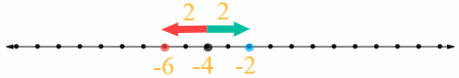First sum is – 2 because it is 2 units left from 0 on the number line.
Second sum is – 6 because it is 6 units left from 0 on the number line.

To find these sums, start from – 4 on the number line and move 2 units to the right to represent the first sum, and 2 unit to the left to represent the second sum.
The first sum is – 2 and the second sum is – 6.

Find each sum.

Question 16.
– 15 + 71 = ______________
Find absolute values and subtract lesser absolute value from greater:
|71| – |- 15| = 56
The final result would be 56 or – 56, depending on signs of given integers.
To find the final result use the sign of number with greater absolute value:
– 15 + 71 = 56

The sum is 56.
Find absolute values, subtract them and add minus if the integer with the greater absolute value was negative.

Question 17.
– 53 + 45 = ______________
Find absolute values and subtract lesser absolute value from greater:
|- 53| – |- 45| = 8
The final result would be 8 or – 8, depending on signs of given integers.
To find the final result use the sign of number with greater absolute value:
– 53 + 45 = – 8

The sum is – 8.
Find absolute values, subtract them and add minus if the integer with the greater absolute value was negative.

Question 18.
– 79 + 79 = ______________
The opposite of an integer is called its additive inverse.
The sum of an integer and its additive inverse is 0
so the final result is
– 79 + 79 = 0

The sum is 0 because the sum of an integer and its additive inverse is 0.

Question 19.
– 25 + 50 = ______________
Find absolute values and subtract lesser absolute value from greater:
|50| – |- 25| = 25
The final result would be 25 or – 25, depending on signs of given integers.
To find the final result use the sign of number with greater absolute value:
– 25 + 50 = 25

The sum is 25.
Find absolute values, subtract them and add minus if the integer with the greater absolute value was negative.

Question 20.
18 + (- 32) = ______________
Find absolute values and subtract lesser absolute value from greater:
|- 32| – |18| = 14
The final result would be 14 or – 14, depending on signs of given integers.
To find the final result use the sign of number with greater absolute value:
18 + (- 32) = – 14

The sum is – 14.
Find absolute values, subtract them and add minus if the integer with the greater absolute value was negative.

Question 21.
5 + (- 100) = ______________
Find absolute values and subtract lesser absolute value from greater:
|- 100| – | 5 | = 95
The final result would be 95 or – 95, depending on signs of given integers.
To find the final result use the sign of number with greater absolute value:
5 + (- 100) = – 95

The sum is – 95.
Find absolute values, subtract them and add minus if the integer with the greater absolute value was negative.

Question 22.
– 12 + 8 + 7 = ______________
First find the sum:
– 12 + 8
Find absolute values and subtract lesser absolute value from greater:
|- 12| – 8 = 4
The result would be 4 or – 4, depending on signs of given integers.
To find the result, use the sign of number with greater absolute value:
– 12 + 8 = – 4
Now, find the sum:
– 4 + 7
Find absolute values and subtract lesser absolute value from greater:
|7| – |- 4| = 3
The final result would be 3 or – 3, depending on signs of given integers.
To find the result, use the sign of number with greater absolute value:
– 4 + 7 = 3
The final result is 3.
The sum is 3.
First, find the sum – 12 + 8 (find absolute values, subtract them and minus if the integer with the greater absolute value was negative) and then add 7 to that sum.

Question 23.
– 8 + (- 2) + 3 = ______________
First find the sum:
– 8 + (- 2)
Find absolute values of given integers:
|- 8| = 8 and |- 2| = 2
Then, sum that absolute values
8 + 2 = 10
The result would be 10 or – 10, depending on sign of given integers (both positive then +, both negative then -)
Given integers are negative so the result is – 10.
Now, find the sum:
– 10 + 3
Subtract the lesser absolute value from the greater:
|- 10| – |3| = 7
The final result would be 7 or – 7, depending on signs of given integers.
To find the result, use the sign of the number with the greater absolute value:
– 10 + 3 = – 7
The final result is – 7.
The sun is – 7
First, find the sum – 8 + (- 2) (find absolute values, sum them and add minus if all integers were negative) and then add 3 to that sum (find absolute values, subtract them and add minus if the integer with the greater absolute value was negative).

Question 24.
15 + (- 15) + 200 = ______________
First, find the sum:
15 + (- 15)
The sum is 0 because
the sum of a number and its additive inverse is 0
Now, find the sum:
0 + 200
The final result is 200.
The sum is 200
First, find the sum 15 + (-15) (the sum of 15 and its additive inverse is 0) and then add 200 to that sum.

Question 25.
– 500 + (- 600) + 1200 = ______________
First, find the sum:
– 500 + (- 600)
Find absolute values of given integers:
|- 500| = 500 and |- 600| = 600
Then, sum that absolute values
500 + 600 = 1100
The result would be 1100 or – 1100, depending on sign of given integers (both positive then +, both negative then -).
Given integers are negative so the result is – 1100
Now, find the sum:
– 1100 + 1200
Subtract the lesser absolute value from the greater:
|1200| – |- 1100| = 100
The final result would be 100 or – 100, depending on signs of given integers
To find the result, use the sign of the number with the greater absolute value:
– 1100 + 1200 = 100
The final result is 100.
The sun is 100
First, find the sum – 500 + (- 600) (find absolute values, sum them and add minus if all integers were negative) and then add 1200 to that sum (find absolute values, subtract them and add minus if the integer with the greater absolute value was negative).

Question 26.
A football team gained 9 yards on one play and then lost 22 yards on the next. Write a sum of integers to find the overall change in field position. Explain your answer.
You can represent the change in field position by writing the sum:
9 + (- 22)
9 shows gain on one play and adding – 22 shows loss on the next
Now, to find the overall change, subtract lesser absolute value from greater:
|- 22| – |9| = 13
Final result would be 13 or – 13, depending on signs of integers.
Since the number with the greater absolute value is – 22, final result is:
9 + (- 22) = – 13

Gain on one play is represented with positive integer 9 and loss on the next game is represented with negative integer – 22 so the sum is 9 + (- 22).
The overall change in field position after two plays is – 13.

Question 27.
A soccer team is having a car wash. The team spent $55 on supplies. They earned$275, including tips. The team’s profit is the amount the team made after paying for supplies. Write a sum of integers that represents the team’s profit.
A soccer team spout $55 on supplies and earned$275 so the sum that represents the team’s profit is:
– 55 + 275
Find absolute values and subtract lesser absolute value from greater:
|275| – |- 55|= 220
Final result would be 220 or – 220. depending on sighs of given integers.
Since 275 ix integer with greater absolute value, the sum is:
– 55 + 275 = 220

The sum that represents soccer team’s profit is – 55 + 275 and the profit is $220. Find absolute values, subtract them and add minus if integer with greater absolute value was negative. Question 28. As shown in the illustration, Alexa had a negative balance in her checking account before depositing a$47.00 check. What is the new balance of Alexa’s checking account? What property did you use to find the sum?The new balance in Alexa’s account is the sum of:
– 47 + 47
Since 47 is additive inverse of – 47 and the sum of integer and its additive inverse is 0, new balance in her account is:
– 47 + 47 = 0

New balance of Alexa’s account is 0.
A property that is used to find the sum is the Inverse Property of Addition.

Question 29.
The sum of two integers with different signs is 8. Give two possible integers that fit this description.
Two possible integers with different signs whose sum is 8 are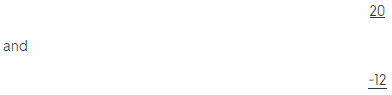You can check it:
Find absolute values and subtract lesser absolute value from greater:
|20| – |- 12| = 8
Final result would be 8 or – 8, depending on signs of given integers.
To find the sum, use the sign of number with greater absolute value:
20 + (- 12) = 8

Two possible integers with different signs whose sum is 8 are 20 and – 12.
Find absolute values, subtract them and add minus it the integer with the greater absolute value was negative.

Question 30.
Multistep Bart and Sam played a game in which each player earns or loses points in each turn. A player’s total score after two turns is the sum of his points earned or lost. The player with the greater score after two turns wins. Bart earned 123 points and lost 180 points. Sam earned 185 points and lost 255 points. Use a problem-solving model to find which person won the game. Explain.
Bait’s total score is the sum:
123 + (- 180)
To find his score, subtract lesser absolute value from greater:
|- 180| – |123| = 57
Final result would be 57 or – 57, depending on signs of given integers.
Use the sign of number with greater absolute value to find the result:
123 + (- 180) = – 57
Bait’s total score after two turns is – 57 points

Sam’s total score is the sum:
185 + (- 255)
To find his score, subtract lesser absolute value from greater:
|- 255| – |185| = 70
Final result would be 70 or – 70, depending on signs of given integers.
Use the sign of number with greater absolute value to find the result:
185 + (- 255) = – 70
Sam’s total score after two turns is – 70 points.

Since both Bart and Sam have negative score after two turns, winner is the player who lost less points.
Bart lost 5 points and Sam lost 70 points so Bart won the game.

H.O.T. FOCUS ON HIGHER ORDER THINKING

Question 31.
Critical Thinking Explain how you could use a number line to show that – 4 + 3 and 3 + (- 4) have the same value. Which property of addition states that these sums are equivalent?
Use number line to represent sums:
– 4 + 3 and 3 + (- 4)
First graph number line with units.
To find first sum, start at – 4 and move 3 units to the right to represent adding 3 (yellow arrow).
To find second sum, start at 3 and move 4 units to the left to represent adding – 4 (red arrow).
Read both sums from number line
The Commutative Property states that these sums are equivalent

Number line:First sum is -1 because it is 1 unit left from 0 on the number line.
Second sum is -1 because it is 1 unit left from 0 on the number line.

Question 32.
Represent Real-World Problems Jim is standing beside a pool. He drops a weight from 4 feet above the surface of the water in the pool. The weight travels a total distance of 12 feet down before landing on the bottom of the pool. Explain how you can write a sum of integers to find the depth of the water.
Jim drops a weight from 4 feet above the surface and it travels 12 feet down until landing on the bottom so the sum that represents the depth of the water is:
4 + (- 12)
To calculate the sum, subtract lesser absolute value from greater:
|- 12| – |4| = 8
Result would be 8 or – 8, depending on signs of given integers
Use the sign of number with greater absolute value to find result:
4 + (- 12) = – 8
Since you want to find the depth of the water, use the absolute value of the result because the depth of the water is positive number. The depth of the water is 8 feet.

Sum that represents the depth is 4 + (-12).
The absolute value of this sum is the depth of the water and that would be 8 feet

Question 33.
Communicate Mathematical Ideas Use counters to model two integers with different signs whose sum is positive. Explain how you know the sum is positive.
Yellow counters represent positive integer and red counters represent negative integer.
To model positive sum of two integers with different sign, take some yellow and some red counters and let there be more yellow counters.
Form and eliminate zero pairs.
When you remove zero pairs, there are some yellow counters left so the sum is positive.

For example:There are more yellow than red counters When you remove zero pairs, there would be some yellow counters left so the sum is positive.

Question 34.
Analyze Relationships You know that the sum of – 5 and another integer is a positive integer. What can you conclude about the sign of the other integer? What can you conclude about the value of the other integer? Explain.